Next: Qualitative Interaction Up: Evaluation Previous: Evaluation

## Quantitative Prediction

For a quantitative measure, the system was trained as usual on the interaction between the two individuals and learned the usual predictive mapping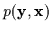. Since real-time is not an issue for this kind of test, the system was actually permitted to use more Gaussian models and more dimensions to learn.

Once trained on a portion of the data, the system's ability to perform prediction was tested on the remainder of the sequence. Once again, the pdf allows us to compute an estimated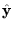for any given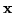short term memory. The expectation was used to predictand not the arg max since it is the least squares estimator. The prediction was then compared to the true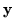result in the future of the time series and RMS errors were computed. Of course, the system only observed the immediate past reaction of both user A and user B which is contained in. Thus, the values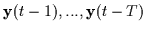are effectively being used to compute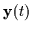. In addition, the system is predicting the immediate reaction of both users (A and B) in the wholevector. For comparison, RMS errors are shown against the nearest neighbour and constant velocity estimates. The nearest neighbour estimate merely assumes that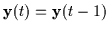and the constant velocity assumes that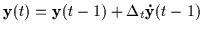.

Table 9.2: RMS Errors
 Nearest Neighbour Constant Velocity ARL 1.57 % 0.85 % 0.62 %

Table 9.2 depicts the RMS errors on the test interaction and these suggest that the system is a better instantaneous predictor than the above two methods. Therefore, it should be useful as a Kalman filter-type of predictor for helping tracking systems.Next: Qualitative Interaction Up: Evaluation Previous: Evaluation
Tony Jebara
1999-09-15